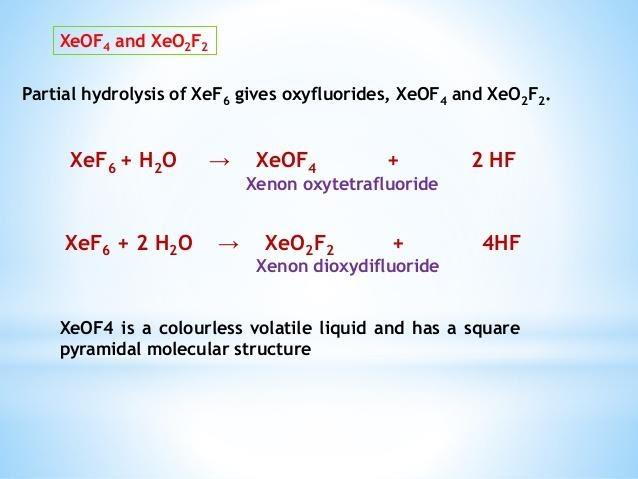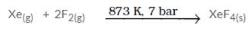Courses

# Test: Group 18 Elements

## 20 Questions MCQ Test Chemistry Class 12 | Test: Group 18 Elements

Description
This mock test of Test: Group 18 Elements for JEE helps you for every JEE entrance exam. This contains 20 Multiple Choice Questions for JEE Test: Group 18 Elements (mcq) to study with solutions a complete question bank. The solved questions answers in this Test: Group 18 Elements quiz give you a good mix of easy questions and tough questions. JEE students definitely take this Test: Group 18 Elements exercise for a better result in the exam. You can find other Test: Group 18 Elements extra questions, long questions & short questions for JEE on EduRev as well by searching above.
*Multiple options can be correct
QUESTION: 1

### Partial hydrolysis of XeF6 yields:

Solution:

C and D are the correct answers.QUESTION: 2

### The valency of inert gas is:

Solution:

The correct answer is Option A.

Valency of an element is defined as its tendency to accept valence electrons.
Since noble gas has completely filled valence (outer) shells, they have the least reactivity.
Hence noble gases are already stable in its elemental form. So its valency is zero.

QUESTION: 3

### When Xenon reacts with fluorine in a ratio of 1:5 at a temperature of 873 K it forms:

Solution:

Xenon reacts with fluorine at 873 K and 7 bar to form XeF4. In this reaction, the ratio of Xenon and fluorine required is 1:5QUESTION: 4

Which one of the following has a linear shape?

Solution:

The correct answer is Option B.

Xe has an electronic configuration of 5s25p6
In XeF2, there are two bonding pairs and three lone pairs of electrons. So acc. to VSEPR theory, the structure would be trigonal bipyramidal to avoid electron pair repulsions.(sp3d)
In XeF4, the structure would be square planar. There are four pairs of bonding electrons and two lone pairs in the molecule. The hybridisation would be sp3d2
XeOF4 has an octahedral geometry due to sp3d2 hybridization. One trans position is occupied by a lone pair giving pyramid shape to the molecule.
Thus  XeF2 has sp3d hybridization.

QUESTION: 5

Which of the following gaseous molecule is monoatomic?

Solution:

Cl, O, and N forms Cl2, O2 and N2 respectively to satisfy octet rule but He has noble gas configuration so do not form any compound and exist as monoatomic gas.

QUESTION: 6

Maximum number of compounds are known in case of :

Solution:

The correct answer is option D
Xe forms maximum no. of compounds among noble gases. It forms bonds with oxygen and fluorine.

QUESTION: 7

Maximum number of compounds are known in the case of which inert gas?

Solution:

The correct answer is Option B
Xe forms maximum no. of compounds among noble gases. It forms bonds with oxygen and fluorine.

QUESTION: 8

The element having highest ionisation potential is:

Solution:

The amount of energy required to remove an electron from the atom decreases. Ionization potential, thus, decreases down a group. The element with highest ionization potential in the periodic table is Helium (2372.1 kj/mol), while the element with the lowest ionization potential is Caesium (375.7 kj/mol).

QUESTION: 9

Noble gases are inert and do not form compounds like other elements because of their:

Solution:

The correct answer is Option C.

Noble gases have completely filled the outer shell/valence shell. Due to this reason they are very stable and do not react with other elements. Hence noble gases are called inert gases means they do not react with other elements to form chemical compounds.

QUESTION: 10

The inert gases have electronic configuration of:

Solution:

The element present in the 18th group (except Helium(He) has the general electronic configuration ns2np6. The elements of the 18th group are called noble gas, having 8 electrons in valence shells, and their outer shells are filled with valence electrons, making them very stable.

QUESTION: 11

Shape of XeF2 is:

Solution:

XeF2 molecular geometry is linear. It acquires such shape as the lone pairs present around the central atom tend to take up equatorial positions. The bond angle is said to be 180°.

QUESTION: 12

Which of the following is the life saving mixture for an asthma patient ?

Solution:

Option C is the correct answer
Mixture of helium and oxygen (density 2) is lighter than air (density 14.4). Hence, it diffuses more rapidly through constricted lung passages.
Mixtures of helium and oxygen are used as life saving mixtures for an asthma patient.

QUESTION: 13

The element which has not yet been reacted with F2 is :

Solution:

The correct answer is option B
The element which has not yet been reacted with F2 is Ar.

QUESTION: 14

Shape of XeF4:

Solution:

The correct answer is Option B
The shape of XeF4​ is square planar. The central Xe atom has 4 bond pairs of electrons and two lone pairs of electrons. It undergoes sp3d2 hybridization which results in octahedral electron geometry and square planar molecular geometry. The two lone pairs are at opposite corners of an octahedron. This minimizes repulsion between them.

QUESTION: 15

In case of noble gases the general trend in the melting points and boiling points, down the group, is

Solution:

The van der Waals force increases with the increase in the size of the atom, and therefore, in general, the boiling and melting points increase from He to Rn. ... Similarly, because of increased dispersion forces, the boiling and melting points of monoatomic noble gases increase from helium to radon.

QUESTION: 16

Which of the outer electronic configuration represent argon?

Solution:

The correct answer is option D
Outer electronic configuration represents argon ns2,np6.
Atomic no. of argon is 18. Its electronic configuration will be
1s2 2s2 2p6 3s2 3p6.
As the outermost shell is full (3s23p6) it is called noble gas.

QUESTION: 17

Geometry of xenon oxyfluoride (XeOF4) is

Solution:
QUESTION: 18

Which among the following Xe compounds is highly explosive and a powerful oxidising agent ?

Solution:

The correct answer is Option A.

XeF6+3H2O→XeO3+6HF
XeF6 is one of the three binary fluorides formed by the xenon. The complete hydrolysis of XeF6 produces XeO3 which is xenon trioxide. This xenon trioxide is highly explosive and acts as a powerful oxidising agent in solution.

QUESTION: 19

Complete hydrolysis of XeF4 and XeF6 results in the formation of:

Solution:

Complete hydrolysis of XeF4 and XeF6 results in the formation of XeO3.
6XeF4 + 12 H2O ----> 2XeO3 + 4Xe + 3O2 + 24HF
XeF6 + 3H2O ----> XeO3 + 6HF

QUESTION: 20

What is the hybridisation of Xe in XeO3?

Solution:

The correct answer is Option A.

In XeO3 , Xe undergoes sp3 hybridisation. sp3 is the molecular geometry of XeO2 which is the trigonal pyramidal. The charge distribution on the central atom is asymmetrical making the molecule polar.